# Community

Blog Events Webinars Tutorials Forum
×
Community Blog Spatial Search: Geometry and GiST Combination Outperforms Geohash and B-tree

# Spatial Search: Geometry and GiST Combination Outperforms Geohash and B-tree

In this article, we explore spatial search performance and describe when to use Geohash, geometry, and geography data types along with indexes such as GiST and B-tree.

By digoal

## Background

PostgreSQL supports three spatial data types: Geohash, geometry, and geography.

1) Geohash is simple, and many databases support it. Geohash takes Earth as a standard sphere, unfolds it as a plane, and divides the plane into several small grids for encoding. Adjacent grids have the same encoding prefix.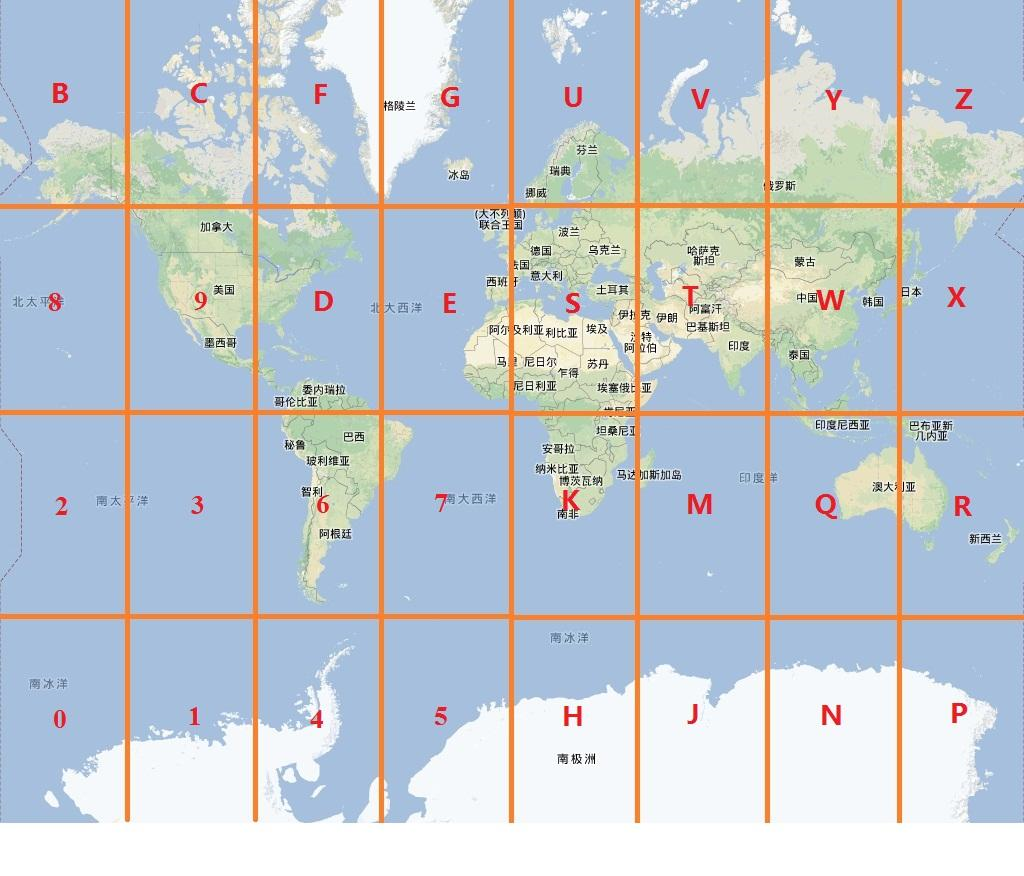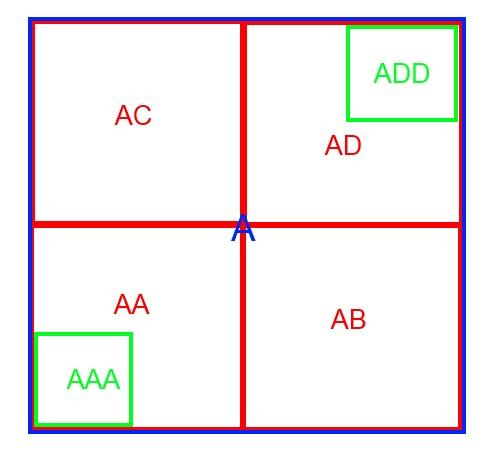The precision of each grid in Geohash is related to the encoding length (based on the assumption that Earth is a standard sphere), as shown below: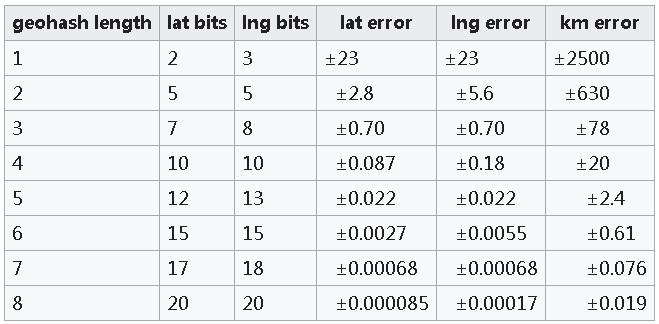2) Earth is neither a standard sphere nor a standard ellipsoid. Therefore, Geohash has an apparent defect in precision, which is solved in geometry and geography.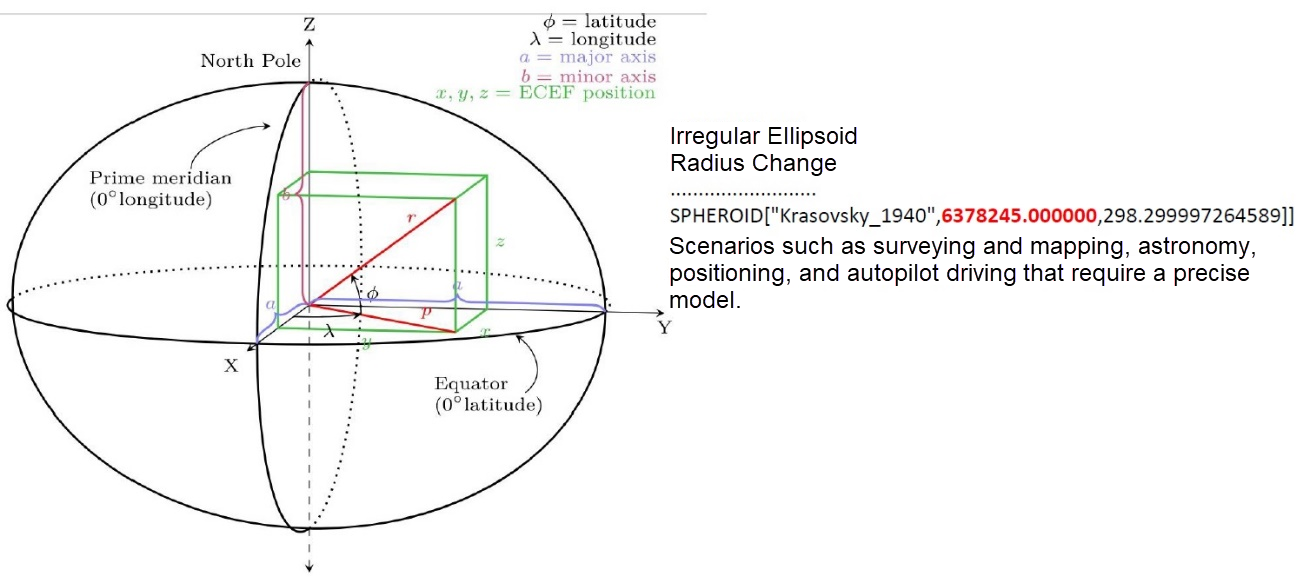In a geographic information system (GIS), two common types of coordinate systems are used: a global or spherical coordinate system (geographical coordinate system) and a two-dimensional coordinate system (projected coordinate system).

The spherical coordinate system is mainly used for calculation, while the two-dimensional coordinate system is primarily used for demonstration.

When you project a spheroid's surface maps onto a plane, you get the projected coordinates of points on the spheroid's surface. Imagine that Earth is a light orb, and you wrap it with a cylinder. After you project Earth's spherical surface maps onto a two-dimensional Cartesian coordinate plane, you get the projected coordinates of the points on Earth's surface on this plane. The larger the projected area, the lower the precision, and the smaller the range.

When calculating the distance between two points, you should consider that they're on a spheroid to get an accurate result.

When it comes to choosing the data type (geometry or geography), we recommend using geometry, which supports both the spherical and the projected coordinate systems. You should select the most suitable coordinate system considering the geographical locations.

The most commonly used coordinate systems are the WGS 84 (SRID=4326) spherical coordinate system and the Mercator projection coordinate system (SRID is EPSG:3857).

Now, let's look at the geometry and geography types. The geometry type supports both planar and spatial objects. However, the geography type only supports spatial objects.

Geometry supports more functions and has a lower cost in terms of geometric calculation.

Geography supports fewer functions and has a higher calculation cost. However, for services across geographical regions, geography is required because its precision is not limited by regions.

Here are some guidelines from PostGIS's website:

• If your data is contained in a small area, you might find that choosing an appropriate projection and using geometry is the best solution in terms of performance and functionality available.
• If your data is global or covers a continental region, you may find that geography allows building a system without worrying about projection details. You store your data in longitude/latitude and use the functions that have been defined on geography.
• If you don't understand projections, don't want to learn about them, and are prepared to accept the functionality limitations in geography, then it might be easier for you to use geography than geometry. Simply load your data up as longitude/latitude and go from there.

Geohash is different in functions and performance from geometry and geography in addition to differences in spatial models.

The encoding precision of Geohash causes some performance problems:

1) Due to Geohash encoding problems, when searching for objects within N meters near a certain point, space amplification will be introduced. In theory, what you need is data within a circle centered on the target point with a radius of N meters.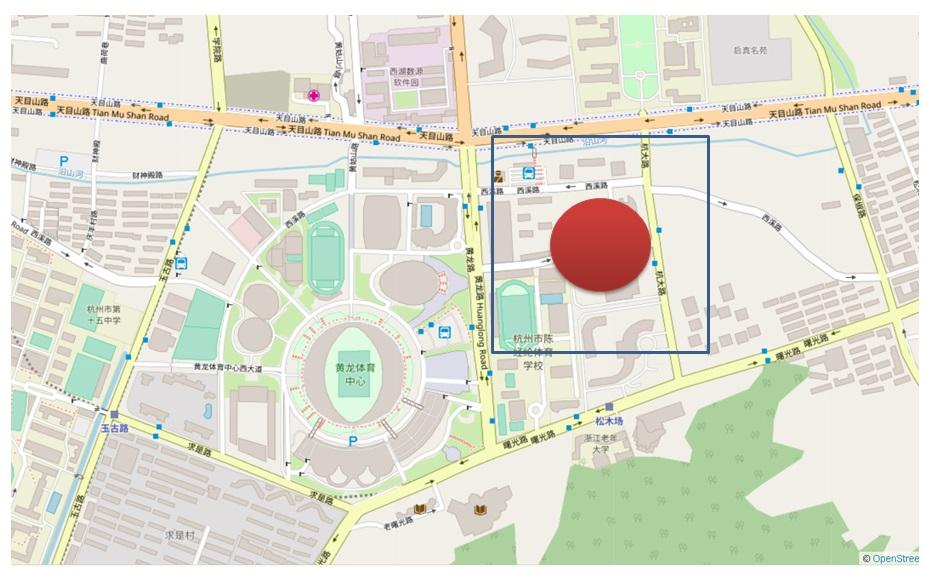If you only look at the prefix, this amplification gets worse when the encoding length becomes shorter.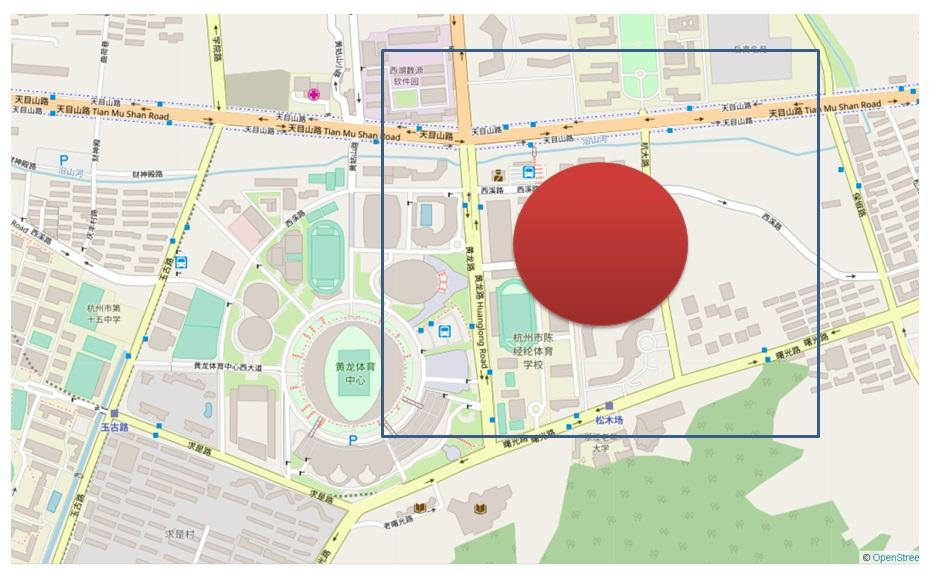Using geometry for distance-based search will not introduce the problem of amplification. If you use a generalized search tree (GiST) index to sort the results by distance and apply the ST_DWithin constraint, only the data inside the circle is returned. You do not need extra recheck filtering.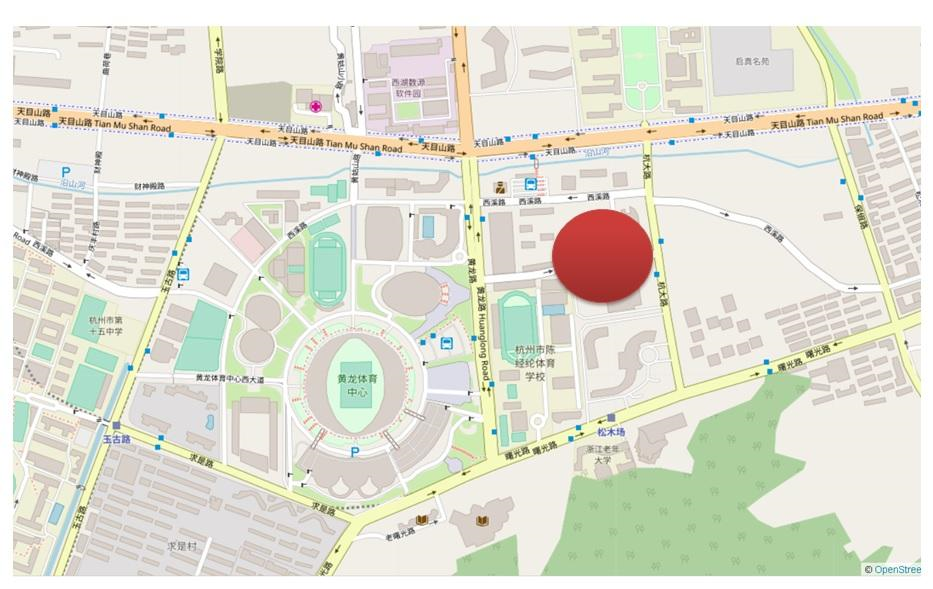Let's look at an example of using the Tantan app at the GIS summit held in Beijing. When you search for 10 restaurants nearby, a small box returns thousands of restaurants in a busy street with a dense point of interest (POI). However, a larger box cannot return even ten restaurants in a remote region.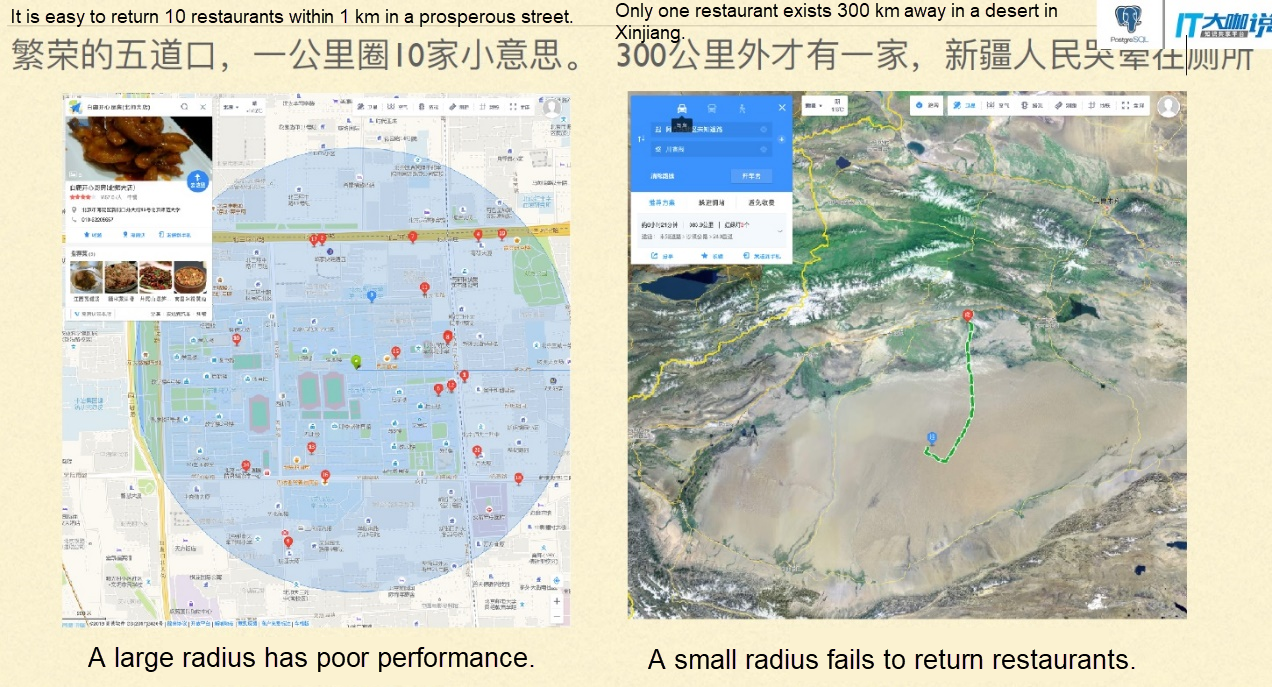2) When you want to search for objects within any polygons, Geohash cannot meet your requirements either. You need to match the objects within a wide range and then perform spatial calculations and filter them one by one.

## Geographic Data Scanning Methods

### 1) Geohash Prefix Scanning

Scans the data in a square block.

``````postgres=# create table t_test(
id int,
pos text,   -- geohash
geo geometry  -- geometry
);
CREATE TABLE

postgres=# insert into t_test
select id,
st_geohash(st_setsrid(st_point(x,y),4326), 13),
st_setsrid(st_point(x,y),4326)
from (
select id, 120+30*random() x, 68+5*random() y
from generate_series(1,100000) t(id)
) t;
INSERT 0 100000
postgres=# select * from t_test limit 10;
id |      pos      |                        geo
----+---------------+----------------------------------------------------
1 | yu0j8y2pxsezp | 0101000020E61000000000625C21F25E400000510228205140
2 | zhsfe7t2cbtzz | 0101000020E6100000008049BE8DBA61400080CB2C5DB15140
3 | zhcydqptr7bkd | 0101000020E6100000000061ED403261400000A01B4B395240
4 | yuhdce4q6u7t6 | 0101000020E610000000808C51B6446040008055F70F005140
5 | yus98nqjtdf4r | 0101000020E610000000803D75C54260400080923722A75140
6 | zk9grxnsqxv98 | 0101000020E61000000000787897A16240008086A312BB5140
7 | yurhhfh33u5xm | 0101000020E61000000080C877DEB96040008031E3B7675140
8 | zhk5qv4vhe10k | 0101000020E610000000002A889E9D61400080CA5360605140
9 | zhm49th6m0h5y | 0101000020E61000000000C79D4DC361400000B456E8575140
10 | zh95n0wvxkpv5 | 0101000020E610000000808F92BE1561400000A9D5FCB55140
(10 rows)
postgres=# create index idx_t_test_1 on t_test (pos text_pattern_ops);
CREATE INDEX
postgres=# explain select * from t_test where pos ~ '^yuhdce4';
QUERY PLAN
-----------------------------------------------------------------------------
Index Scan using idx_t_test_1 on t_test  (cost=0.42..2.64 rows=10 width=50)
Index Cond: ((pos ~>=~ 'yuhdce4'::text) AND (pos ~<~ 'yuhdce5'::text))
Filter: (pos ~ '^yuhdce4'::text)
(3 rows)

postgres=# explain select * from t_test where pos like 'yuhdce4%';
QUERY PLAN
-----------------------------------------------------------------------------
Index Scan using idx_t_test_1 on t_test  (cost=0.42..2.64 rows=10 width=50)
Index Cond: ((pos ~>=~ 'yuhdce4'::text) AND (pos ~<~ 'yuhdce5'::text))
Filter: (pos ~~ 'yuhdce4%'::text)
(3 rows)  ``````

### 2) Geohash Range Scanning

Scans a small grid in a continuous Z space.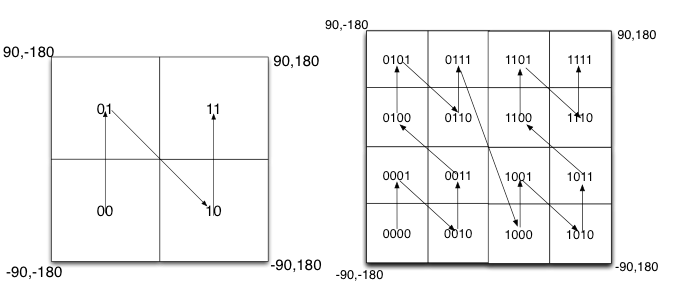Fill the binary encoding results in the space. When the space is divided into four blocks, the encoding order is 00 in the lower-left corner, 01 in the upper-left corner, 10 in the lower-right corner, and 11 in the upper-right corner. This is similar to a Z-shaped curve. When we recursively decompose each block into smaller sub-blocks, the encoding sequence is self-similar (fractal), and each sub-block also forms a Z curve. This type of curve is called Peano's space-filling curve.

``````postgres=# explain select * from t_test where pos ~>=~ 'yuhdce4' and pos ~<=~ 'yuhdcej';
QUERY PLAN
----------------------------------------------------------------------------
Index Scan using idx_t_test_1 on t_test  (cost=0.42..2.64 rows=1 width=50)
Index Cond: ((pos ~>=~ 'yuhdce4'::text) AND (pos ~<=~ 'yuhdcej'::text))
(2 rows)  ``````

### 3) Longitude and Latitude Range Scanning

Separately stores longitudes and latitudes into two fields. You can obtain a rectangular interval that falls within the latitude and longitude range.

``````create table t_geo (id int, x float, y float);

insert into t_geo
select id, 120+30*random() x, 68+5*random() y
from generate_series(1,100000) t(id) ;
postgres=# create index idx_t_geo_1 on t_geo (x,y);
CREATE INDEX

postgres=# explain select * from t_geo where x >= 120 and x <=124 and y >= 68 and y <=71;
QUERY PLAN
-------------------------------------------------------------------------------------------------------------------------------------------------------
Index Scan using idx_t_geo_1 on t_geo  (cost=0.42..1029.31 rows=7810 width=20)
Index Cond: ((x >= '120'::double precision) AND (x <= '124'::double precision) AND (y >= '68'::double precision) AND (y <= '71'::double precision))
(2 rows)  ``````

### 4) Geometry- and GiST-based Spatial Scanning

PostGIS supports GiST-based R-tree spatial indexes. Each layer is a bounding box. You can quickly locate objects that contain, intersect with, or adjacent to an input object.

``create index idx_t_test_2 on t_test using gist (geo);  ``

GiST indexes support not only spatial search but also spatial sorting.

``````postgres=# explain select * from t_test where st_dwithin(geo, st_setsrid(st_point(121, 70), 4326), 10000) order by geo <-> st_setsrid(st_point(121, 70), 4326);
QUERY PLAN
-------------------------------------------------------------------------------------------------------------------------------------------------
Index Scan using idx_t_test_2 on t_test  (cost=0.28..29263.75 rows=6667 width=58)
Index Cond: (geo && '0103000020E6100000010000000500000000000000804BC3C0000000000065C3C000000000804BC3C00000000000ABC3400000000080C4C3400000000000ABC3400000000080C4C340000000000065C3C000000000804BC3C0000000000065C3C0'::geometry)
Order By: (geo <-> '0101000020E61000000000000000405E400000000000805140'::geometry)
Filter: (('0101000020E61000000000000000405E400000000000805140'::geometry && st_expand(geo, '10000'::double precision)) AND _st_dwithin(geo, '0101000020E61000000000000000405E400000000000805140'::geometry, '10000'::double precision))
(4 rows)  ``````

In location-based service (LBS) projects, it is strongly desired to sort the output by distance from near to far.

## Performance Comparison

A GiST index is much faster than a two-field composite index. When the composite index drives the use of a column range, all records in the range are scanned.

## Summary

1) Geohash applies to scenarios that have low precision requirements, for example, local small-range services. It also applies to scenarios insensitive to computing resource consumption. Due to the large granularity, areas returned by the index may have a large amount of invalid data that needs to be rechecked frequently. Besides, Geohash does not support sorting, so additional sorting overhead is required.

2) Geometry and GiST spatial indexes are suitable for scenarios that require high precision and less resource consumption. They are perfect for professional GIS services. Unlike geography, geometry-based searches need to use the correct coordinate system.

3) To search for 100 nearby points in an area with sparse or dense objects, you must select a proper prefix. This could be challenging when you use Geohash. Using geometry and GiST can easily and efficiently solve this problem.

``````select * from tbl where pos ~ '^geohash_多长合适呢? 不知道' limit 100;
select * from tbl order by geo <-> 点 limit 100;  ``````

PostgreSQL supports all Geohash, geometry, and geography data types. You can use the one that best fits your needs.

0 0 0
Share on

# Related Products

• ##Database Overview

Fully managed and less trouble database services

• ##AnalyticDB for PostgreSQL

An online MPP warehousing service based on the Greenplum Database open source program

• ##ApsaraDB RDS for PostgreSQL

An on-demand database hosting service for PostgreSQL with automated monitoring, backup and disaster recovery capabilities

• ##Database for FinTech Solution

Leverage cloud-native database solutions dedicated for FinTech.